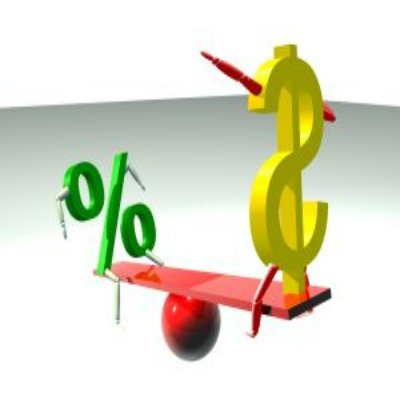# How to Work Out Percentages Increase and Decrease

It may not be wrong to say that when we have to verbally measure something or express its intensity, it is usually done with the word PERCENT involved. Apart from this, we also use the word percent in order to specify a ratio or proportion of something with another entity. For example, when we say that a certain drink has 65% of Alcohol, this indicates the proportion in which alcohol is present in that drink. Moreover, when it comes to solving sums related to percentages, some students find it difficult. However, it is worth mentioning here that working out percentages involves a series of simple steps. This article describes different situations in which percentage is involved.

### Instructions

• 1

Making Results

Percentages have a big role to play when it comes to results. This is because the positions achieved by students depend on the percentage obtained. Hence, the percentages are based on the marks obtained out of the total or maximum marks.

Example 1. A student obtained 880 marks out of the total 1100. Express these marks in terms of a percentage.
Max. Marks = 1100
Marks Obtained = 880
Percentage = 880/1100 x 100
= 80%

Example 2. A student secured 90% marks out to of the total 250. Calculate the marks secured by the student.
Total Marks = 250
% of student = 90
Marks secured = 90/100 x 250
= 225• 2

Calculating Interest

When you borrow an amount from the bank or a financial institute, then you have to pay some amount as interest which is referred as the profit earned by the bank upon offering you the service. The interest is expressed by the banks in terms of a percentage.

Example. A man gets a loan of \$ 400,000 from a bank for 3 years with an annual interest rate of 5%. Calculate the total amount of interest the man will pay in 3 years.

Amount borrowed = \$ 400,000
Tenure = 3 years
Interest rate per annum = 5%
Amount of interest per year = 5/100 x 400,000
= \$ 20,000

Total amount of interest in 3 years = 20,000 x 3
= \$ 60,000• 3

Determining Profit and Loss

The profit and loss is also expressed and calculated in terms of a percentage. You buy something at a certain price and sell it at another price. The difference in the two amounts will be the percentage of a profit or loss.

Example. A man buys a property for \$ 400,000 and sells it for \$ 450,000. Then he buys another property for \$ 450,000 but lost \$ 20,000 upon selling it. Calculate the profit and loss in each case and the net profit.

Cost price of first property = \$ 400,000
Selling price of first property = \$ 450,000
Amount of Profit = Selling Price – Cost price
= 450,000 – 400,000
= 50,000

% of profit = 50,000/ 450,000 x 100
= 11.11 %

Cost price of the second property = \$ 450,000
Selling price of the second property = \$ 450,000 - \$ 20,000
= 430,000

% of loss = 20,000/450,000 x 100
= 4.44 %

Net profit = 11.11 – 4.44
= 6.7 % of \$ 400,000

•